# Counting Rows that Match Specific Criteria for Each Column in Microsoft Excel 2010

In this article, we will learn how to count rows that match specific criteria for each column.

If you want to count the rows that match specific criteria for each column, you can use SUMPRODUCT function to get the output.

SUMPRODUCT: Returns the sum of the products of corresponding ranges or arrays.

Syntax: =SUMPRODUCT(array1,array2,array3,...)

array1,array2,….. array255 are the ranges of cells or arrays that you want to multiply & then add them.

There can be maximum 255 arrays in Sumproduct function.Refer below shown screenshot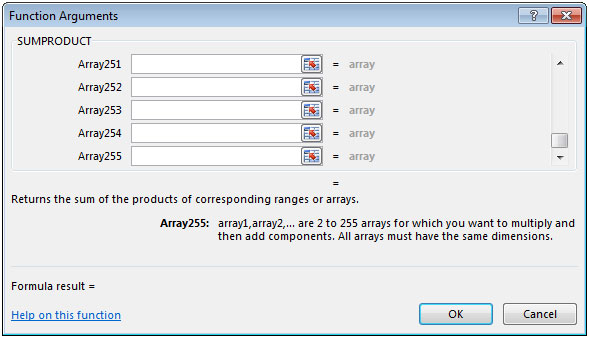Let us take an example:

• We have Employee data i.e. ID in column A, Gender in column B & Age of Employee in column C.
• Two criteria are defined in cells E2 & F2 as Male & Age of 25 years
• In cell F6, the result needs to be calculated.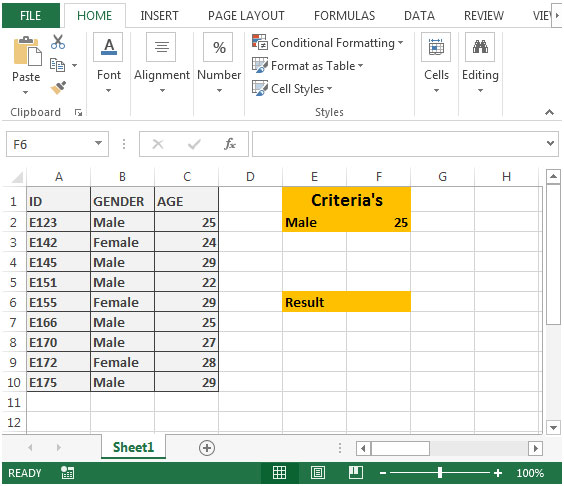• In cell F6, the formula would be =SUMPRODUCT((B2:B10=E2)*(C2:C10=F2))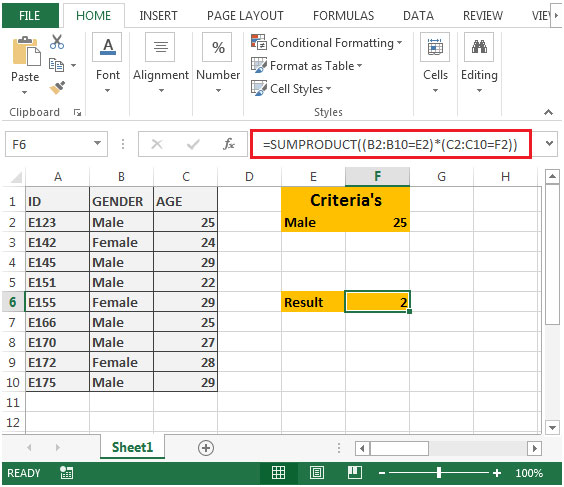• If we evaluate the formula “B2:B10=E2” by selecting first & then press F9 shortcut key for evaluation; we will get the following string of TRUE & FALSE
{TRUE;FALSE;TRUE;TRUE;FALSE;TRUE;TRUE;FALSE;TRUE}
• When we evaluate “C2:C10=F2”, we will get the following string of TRUE & FALSE
{TRUE;FALSE;FALSE;FALSE;FALSE;TRUE;FALSE;FALSE;FALSE}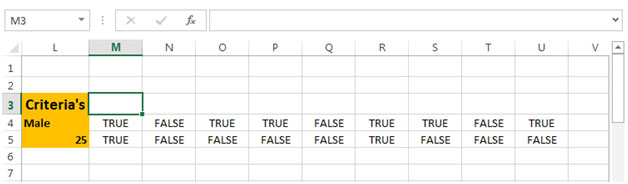• If we multiply the string of TRUE & FALSE with each other like shown in below screenshot, we will get either 1 or 0 as output.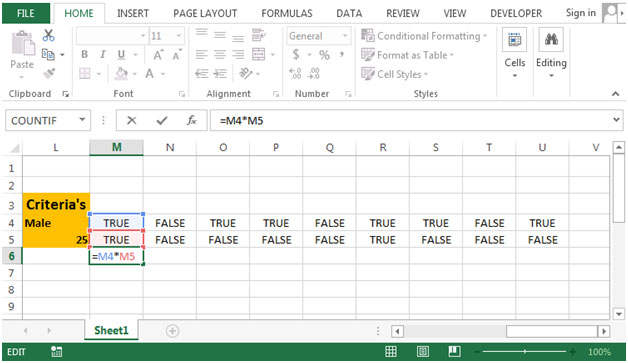• Copying the formula from cell M6 to range N6:U6, we will get the desired result.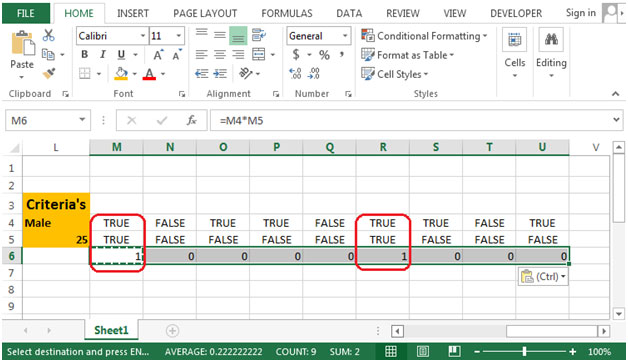• In above shown screenshot, we have only 2 results that are matching the criteria. Hence, the output will be 2.

Terms and Conditions of use

The applications/code on this site are distributed as is and without warranties or liability. In no event shall the owner of the copyrights, or the authors of the applications/code be liable for any loss of profit, any problems or any damage resulting from the use or evaluation of the applications/code.